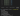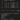# How to Format Bytes to KB, MB, GB, TB or PB

## Problem#

When you execute a script, sometimes data or file size is not represented as KB, MB, GB, etc. Instead the result only shows the number.

For example, you want to get disk size and free space for all drives in your computer using WMI script as follows:

``````
Get-WmiObject -ClassName Win32_LogicalDisk | ForEach-Object {
[PSCustomObject]@{
DeviceID  = \$_.DeviceID
Size      = \$_.Size
FreeSpace = \$_.FreeSpace
}
}

``````

By default the result will only shows the number as follows:It would be more intuitive if the number is formatted as other unit in Byte like KB, MB, GB, etc.

## Solution#

In PowerShell, there are 5 byte units to represent large byte values:

• KB: Kilobytes (1024^1)
• MB: Megabytes (1024^2)
• GB: Gigabytes (1024^3)
• TB: Terabytes (1024^4)
• PB: Petabytes (1024^5)

### Using division by 1 and format operator#

To convert Byte to KB, MB, GB, etc,. we can divide the number with 1 as follows:

``````
509238833152 / 1KB
509238833152 / 1MB
509238833152 / 1GB
509238833152 / 1TB
509238833152 / 1PB

``````

Below is the output:To make it more readable, we can format above numbers using format operator so that there will be separator (typically comma or dot) for every three digits from the last integer part as well as 2 decimal places for floating part as follows:

``````
\$number = 509238833152

'{0:N2} KB' -f (\$number / 1KB)
'{0:N2} MB' -f (\$number / 1MB)
'{0:N2} GB' -f (\$number / 1GB)
'{0:N2} TB' -f (\$number / 1TB)

``````

Below is the output:## Conclusion#

In conclusion, to format number to other Byte units like KB, MB, GB, TB, PB, we can use division by 1 and specify the unit. For comma or dot formatting, we can use format operator `-f`.